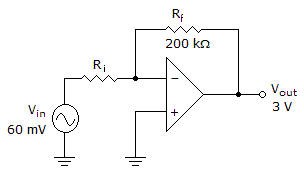# Electronics - Operational Amplifiers - Discussion

### Discussion :: Operational Amplifiers - General Questions (Q.No.10)

10.Decreasing the gain in the given circuit could be achieved by

 [A]. reducing the amplitude of the input voltage [B]. increasing the value of the feedback resistor [C]. increasing the value of the input resistor [D]. removing the feedback resistor

Explanation:

No answer description available for this question.

 Uuumkl said: (Mar 3, 2011) In inverting amp gain is-rf/ri if we decrease gain ri will increase.

 Vatsala Sharma said: (May 23, 2011) Gain of inverting amplifier is inversely proportional to input resistance. So, gain decreases when input resistance is increase.

 Manoj S Awakhare said: (Jun 30, 2012) Acctually the diagram is in inverting configuration. So it has gain equation Gain= -Rf/Rin. It means gain inversly proportional to Rin. Therefore answer is C.

 Rashmi said: (Aug 12, 2012) Gain of an inverting amplifier= -Rf/Ri, therefore the answer is "C".

 Dushyanth said: (Jan 11, 2015) Why can't removing the feedback resistor be an option. Since Gain = Rf/Ri. And Rf = ohms if we remove the feedback resistor Rf = 0. So Rf is reduced so gain will also be reduced. But why is't that a correct option.

 Shahid said: (Feb 12, 2015) @Dushyanth. Removing feedback resistor will make Rf = Infinity whereas shorting it will make feedback resistor Rf=0.